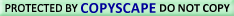HUNGRY? TRY comCustom Search

Hey, you're in the power supply zone--YOUR HANDYMAN ZONE!

Power Supply 1-2-3: Direct Current (DC)

# DC NETWORK ANALYSIS

## Introduction to network theorems

Anyone who's studied geometry should be familiar with the concept of a theorem: a relatively simple rule used to solve a problem, derived from a more intensive analysis using fundamental rules of mathematics. At least hypothetically, any problem in math can be solved just by using the simple rules of arithmetic (in fact, this is how modern digital computers carry out the most complex mathematical calculations: by repeating many cycles of additions and subtractions!), but human beings aren't as consistent or as fast as a digital computer. We need �shortcut� methods in order to avoid procedural errors.

In electric network analysis, the fundamental rules are Ohm's Law and Kirchhoff's Laws. While these humble laws may be applied to analyze just about any circuit configuration (even if we have to resort to complex algebra to handle multiple unknowns), there are some �shortcut� methods of analysis to make the math easier for the average human.

As with any theorem of geometry or algebra, these network theorems are derived from fundamental rules. In this chapter, I'm not going to delve into the formal proofs of any of these theorems. If you doubt their validity, you can always empirically test them by setting up example circuits and calculating values using the �old� (simultaneous equation) methods versus the �new� theorems, to see if the answers coincide. They always should!Use of/Visit to this site constitutes the User's Understanding of and Consent to  | Privacy Policy All links found on this site should be considered as Ad links.  See Ad Disclosures for more details.Monitored for Copyright Compliance